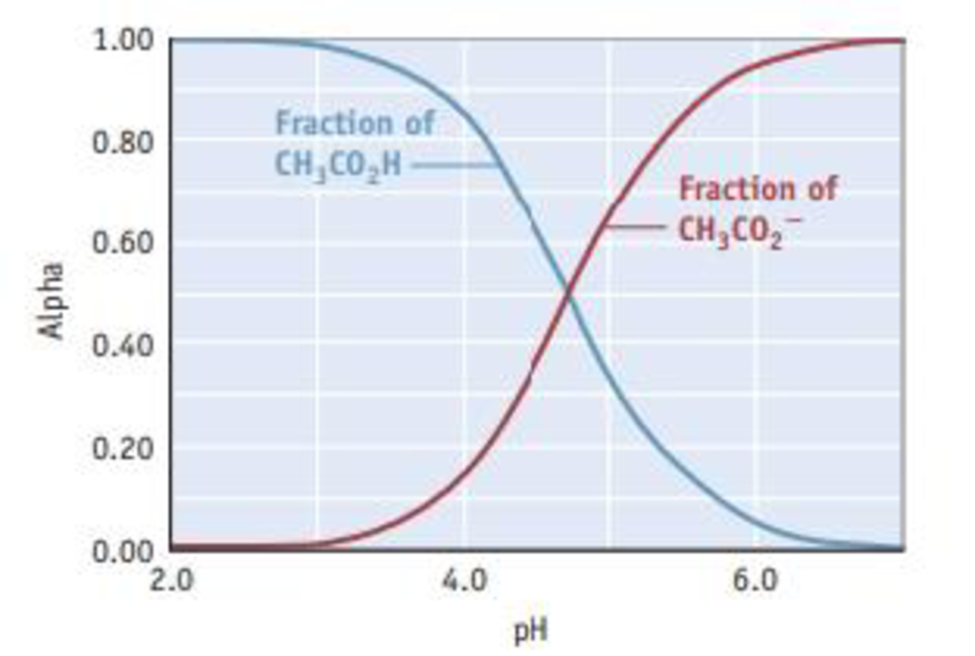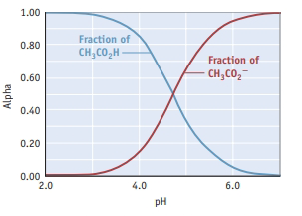Chapter 17, Problem 117SCQ

Chapter
Section
Textbook Problem

Composition diagrams, commonly known as “alpha plots,” are often used to visualize the species in a solution of an acid or base as the pH is varied. The diagram for 0.100 M acetic acid is shown here.The plot shows how the fraction [alpha (α)] of acetic acid in solution, α = [ C H 3 C O 2 H ] [ C H 3 C O 2 H ] + [ C H 3 C O 2 − ] changes as the pH increases (blue curve). (The red curve shows how the fraction of acetate ion, CH3CO2−, changes as the pH increases.) Alpha plots are another way of viewing the relative concentrations of acetic acid and acetate ion as a strong base is added to a solution of acetic acid in the course of a titration. (a) Explain why the fraction of acetic acid declines and that of acetate ion increases as the pH increases. (b) Which species predominates at a pH of 4, acetic acid or acetate ion? What is the situation at a pH of 6? (c) Consider the point where the two lines cross. The fraction of acetic acid in the solution is 0.5, and so is that of acetate ion. That is, the solution is half acid and half conjugate base; their concentrations are equal. At this point, the graph shows the pH is 4.74. Explain why the pH at this point is 4 74.

a)

Interpretation Introduction

Interpretation:

The following by using the given  pH plot has to be determined.1. (a) Explain why the fraction of acetic acid declines and that of acetic acid that of acetate ion increases as the pH increase.

Concept introduction:

The given plot shows how the fraction (α) of acetic acid solution.

α = [CH3CO2H][CH3CO2H]+[CH3CO2-]

It changes as the pH increases (blue curve).(The red curve shows how the fraction of acetate ion, CH3CO2- changes as the pH increase).Alpha plots are another way of viewing the relative concentrations of acetic acid and acetate ion as a strong base is added to a solution of acetic acid in the course of a titration.

Explanation

The value of pKa of acetic acid is 4.74. The pKa value of an acid becomes predominately deprotonated by increasing the pH of an acid.

This is because of the forward reaction of the dissociation equilibrium is more favoured. It leads to [H3O+

b)

Interpretation Introduction

Interpretation:

The following by using the given  pH plot has to be determined.Which species predominate at a pH of 4, acetic acid or acetate ion? What is the situation at a pH of 6?

Concept introduction:

The given plot shows how the fraction (α) of acetic acid solution.

α = [CH3CO2H][CH3CO2H]+[CH3CO2-]

It changes as the pH increases (blue curve).(The red curve shows how the fraction of acetate ion, CH3CO2- changes as the pH increase).Alpha plots are another way of viewing the relative concentrations of acetic acid and acetate ion as a strong base is added to a solution of acetic acid in the course of a titration.

c)

Interpretation Introduction

Interpretation:

The following by using the given  pH plot has to be determined.Consider the point where the two lines cross. The fraction of acetic acid in the solution is 0.5, and so is that of acetate ion. That is the solution i half acid and half conjugate base. Their concentrations are equal. At this point, the graph shows the pH is 4.74. Explain the pH at this point is 4.74.

Concept introduction:

The given plot shows how the fraction (α) of acetic acid solution.

α = [CH3CO2H][CH3CO2H]+[CH3CO2-]

It changes as the pH increases (blue curve).(The red curve shows how the fraction of acetate ion, CH3CO2- changes as the pH increase).Alpha plots are another way of viewing the relative concentrations of acetic acid and acetate ion as a strong base is added to a solution of acetic acid in the course of a titration.

Still sussing out bartleby?

Check out a sample textbook solution.

See a sample solution

The Solution to Your Study Problems

Bartleby provides explanations to thousands of textbook problems written by our experts, many with advanced degrees!

Get Started